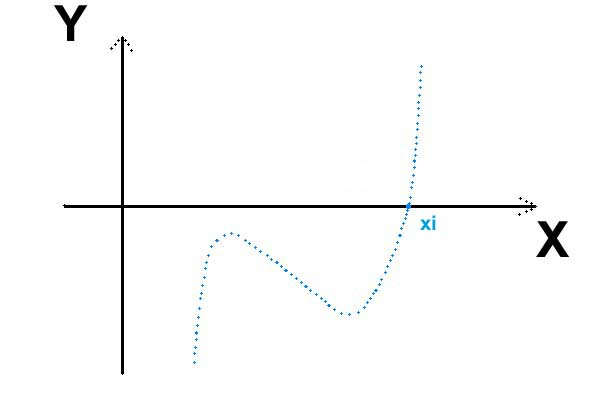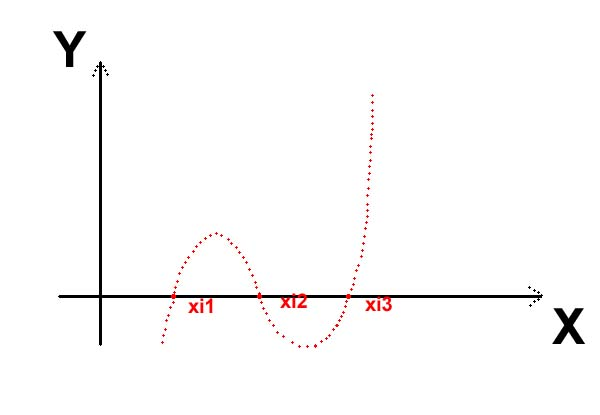# Cubic Number Fields

## Structures that are Generated by Irreducible Polynomials of the 3rd Degree with Integer Coefficients

Polynomials of the 3rd Degree:

Any polynomial of the 3rd degree can be freed from the quadratic term by a TSCHIRNHAUS transform. (Such transforms have first been studied systematically by Graf Ehrenfried Walter von TSCHIRNHAUSEN from Kieslingswalde bey Görlitz.) Hence we can assume without loss of generality that the polynomial has the normal form P(X) = X3 + CX + D with rational integer coefficients C and D. A zero xi of a polynomial of the 3rd degree, which is irreducible over the field Q of rational numbers, generates a cubic number field L = Q(xi) by adjoining xi to Q. According to the number of real zeros of the generating polynomial P(X), we distinguish simply real (or complex) cubic fields and totally real cubic fields.

1. Simply Real Cubic Fields:Over the field R of real numbers, P(X) has a factorization P(X) = (X - xi) (X2 + beta X + gamma) with the real zero xi in R and a polynomial of the 2nd degree with coefficients beta,gamma in R which is irreducible over R. Over the field C of complex numbers, this quadratic factor splits further into (X - xi') (X - xi'') with the conjugate complex zeros xi',xi'' in C. The real cubic number field L = Q(xi) has two conjugate isomorphic complex cubic number fields L' = Q(xi') and L'' = Q(xi''). The field monomorphisms of L consist of a real inclusion L-->R, xi-->xi and a pair of conjugate complex embeddings L-->C, xi-->xi', L-->C, xi-->xi''. The signature (s,t) of L is (1,1), where s denotes the number of real embeddings and t the number of pairs of conjugate complex embeddings. Hence, the Unit Theorem of Dirichlet shows that the unit group U_L of L can be generated by a single fundamental unit e (of infinite order) and the root of unity -1: U_L = < e, -1 >.

2. Totally Real Cubic Fields:P(X) splits completely into linear factors over R, P(X) = (X - xi1) (X - xi2)(X - xi3) with the three real zeros xi1,xi2,xi3 in R. The three zeros generate three conjugate isomorphic real cubic number fields L1 = Q(xi1), L2 = Q(xi2), L3 = Q(xi3). Among the field monomorphisms of L1 we have the real inclusion L1-->R, xi1-->xi1 and the real embeddings L1-->R, xi1-->xi2, L1-->R, xi1-->xi3. Therefore, L1 has the signature (s,t) = (3,0). According to the Unit Theorem of Dirichlet the unit group U_L of L has a fundamental system of exactly 2 units e_1 and e_2 (of infinite order) and the root of unity -1, in this case: U_L = < e_1, e_2, -1 >.

Like any ABELian group, the unit group U_K of an algebraic number field K splits into the direct product of the part of finite order and the part of infinite order. The part of finite order is generally called the torsion subgroup and here specifically the subgroup of the roots of unity or torsion units TU_K. By the DIRICHLET unit rank r we understand the number of free generators of infinite order, i. e., the Z-Rank of the torsion free part. The fundamental Unit Theorem of DIRICHLET claims that the DIRICHLET unit rank r can be calculated from the signature (s,t) in the following manner: r = s + t - 1. (The signature is connected with the absolute degree n = [K:Q] of K by the relation s + 2t = n.)

By a TSCHIRNHAUS transform we understand in the most general case the mapping, which associates the main polynomial H_rho(X) of rho with each element rho of an algebraic number field L: L --> Q[X], rho --> H_rho(X) = det( X*1_L - h_rho ), where h_rho in End_Q(L) denotes the homothety alpha --> rho * alpha. The TSCHIRNHAUS transform is independent from the choice of a Q-basis of L. In particular, it is not affected by the selection of a primitive element delta of L = Q( delta ) with associated power basis (1,delta,...,deltan-1), where n denotes the degree [L:Q] of L over Q. For the actual computation, however, we choose a primitive element delta of L. Then we obtain the impression that the TSCHIRNHAUS transform maps the minimal polynomial M_delta(X) of delta onto the main polynomial H_rho(X) of rho: L * { P(X) in Q[X] | P monic and irreducible of degree n } --> Q[X], ( rho, M_delta(X) ) --> H_rho(X) where rho = r0 + r1*delta + ... + rn-1*deltan-1, since L = Q + Q*delta + ... + Q*deltan-1 as a Q-vectorspace, M_delta(X) = Xn + Mn-1*Xn-1 + ... + M1*X + M0, and H_rho(X) = Xn + Hn-1*Xn-1 + ... + H1*X + H0. The coefficients Hi of the main polynomial H_rho(X) appear as polynomials in the coordinates ri of rho with respect to delta and in the coefficients Mi of the minimal polynomial M_delta(X).